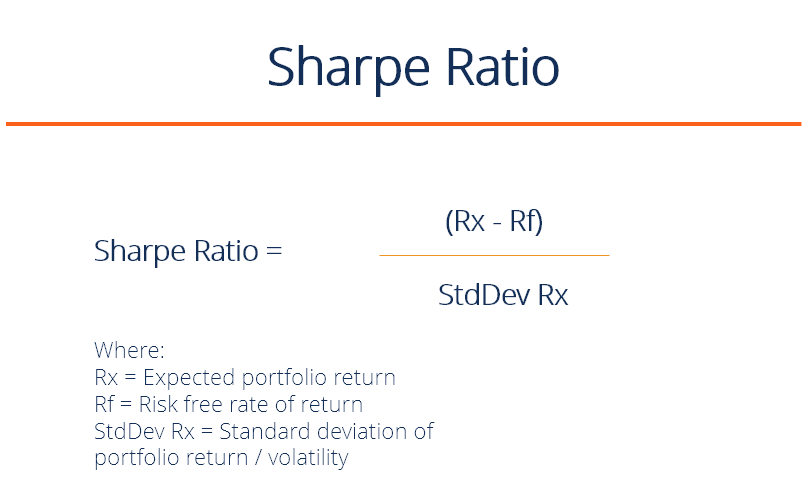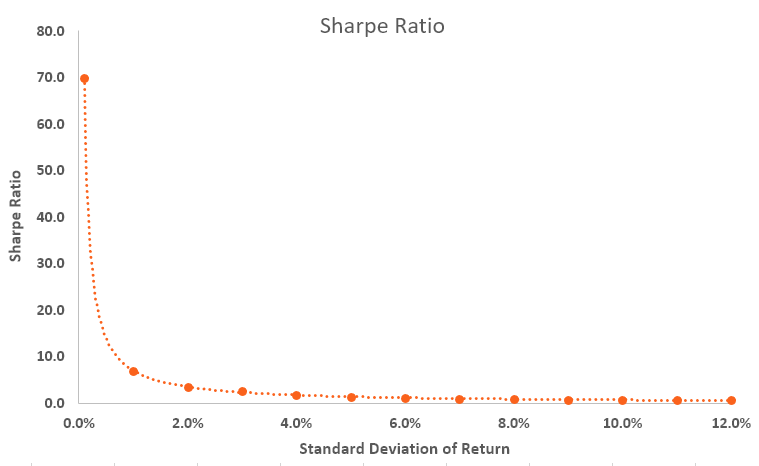# Sharpe Ratio

The golden industry standard for risk-adjusted return

## What is the Sharpe Ratio?

Named after American economist, William Sharpe, the Sharpe Ratio (or Sharpe Index) is commonly used to gauge the performance of an investment by adjusting for its risk.

The higher the ratio, the greater the investment return relative to the amount of risk taken, and thus, the better the investment. The ratio can be used to evaluate a single stock or investment, or an entire portfolio.### Sharpe Ratio Formula

Sharpe Ratio = (Rx – Rf) / StdDev Rx

Where:

• Rx = Expected portfolio return
• Rf = Risk free rate of return
• StdDev Rx = Standard deviation of portfolio return (or, volatility)

• 2 – 2.99: Great
• Greater than 3: Excellent

### What Does It Really Mean?

It’s all about maximizing returns and reducing volatility. If an investment had an annual return of only 10% but had zero volatility, it would have an infinite (or undefined) Sharpe Ratio.

Of course, it’s impossible to have zero volatility, even with a government bond (prices go up and down).  As volatility increases, the expected return has to go up significantly to compensate for that additional risk.

The Sharpe ratio reveals the average investment return, minus the risk-free rate of return, divided by the standard deviation of returns for the investment. Below is a summary of the exponential relationship between the volatility of returns and the Sharpe Ratio.### Application of the Sharpe Ratio

An investment portfolio can consist of shares, bonds, ETFs, deposits, precious metals, or other securities. Each security has its own underlying risk and return levels, which will influence the ratio.

For example, assume that a hedge fund manager has a portfolio of stocks with a ratio of 1.70. The fund manager decides to add some commodities to diversify and modify the composition to 80/20,  stocks/commodities, which pushes the Sharpe ratio up to 1.90.

While the portfolio adjustment might increase the overall level of risk, it pushes the ratio up, thus indicating a more favorable risk/reward situation. If the portfolio change causes the ration to go down, then the portfolio addition, while potentially offering attractive returns, would be evaluated by many financial analysts as carrying an unacceptable level of risk, and the portfolio change would not be made.

### Example of the Sharpe Ratio

Consider two fund managers, A and B. Manager A has a portfolio return of 20% while B has a return of 30%. S&P 500 performance is 10%. Although it looks like B performs better in terms of return, when we look at the Sharpe Ratio, it turns out that A has a ratio of 2 while B’s ratio is only 0.5.

The numbers mean that B is taking on substantially more risk than A, which may explain his higher returns, but which also means he has a higher chance of eventually sustaining losses.

• Accounting Rate of Return (ARR)
• Risk and Return
• Internal Rate of Return (IRR)
• Financial Modeling Guide

### Financial Analyst Training

Get world-class financial training with CFI’s online certified financial analyst training program!

Gain the confidence you need to move up the ladder in a high powered corporate finance career path.

Learn financial modeling and valuation in Excel the easy way, with step-by-step training.